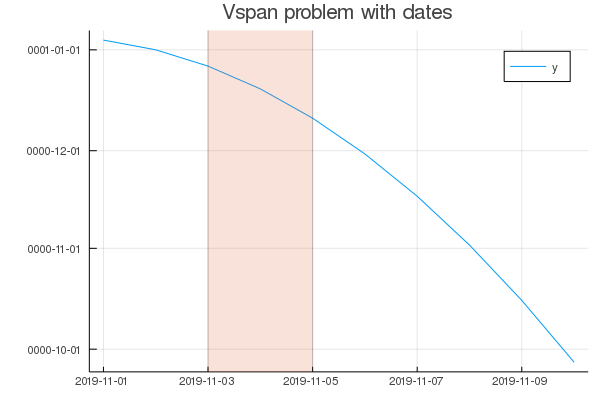# Vspan vertical scale wrong when using dates as x axis

Hello,
I am trying to use vspan to highlight specific periods in a plot with dates as x axis. It seems that vspan changes the y axis units to dates also (which is wrong).

MWE :

``````# Show vspan problem with date abscissaes
using Plots
using Dates

gr()
#ENV["GKSwstype"]="nul" # from batch / no X11
#ext=".svg"
ext=".png"
sf=true

y=[1.0*i*i for i in 1:10]
@. y= -y + 5.0

# x=[i+0.0 for i in 1:10]
x=[Date(2019,11,i) for i in 1:10]

rx=[x,x]
ry=[y,y]

p=plot(x,y, label="y", title="Vspan problem with dates")
if sf; savefig(p,"p-plot"*ext); end

p=vspan!(p,rx,label="",alpha=0.2)
if sf; savefig(p,"p-vspan"*ext); end
``````

Here is the (incorrect ?) resultWhat can I do to go around this ?

1 Like

Maybe file an issue with Plots.jl - I remember having this issue with vline which got fixed a while ago, maybe that fix wasn’t transposed to vspan

Thank you @nilshg ! You are right, the problem was fixed inside vline, while it remains in vspan (and bar ?).
But this gave me the following go-around : if i do an additional vline!, this fixes the y axis scale !``````  p=vline!(p,[rx], label="",alpha=0.0)
``````

So, thank you for helping solve my problem.

1 Like

Haha, nice one, glad that worked!